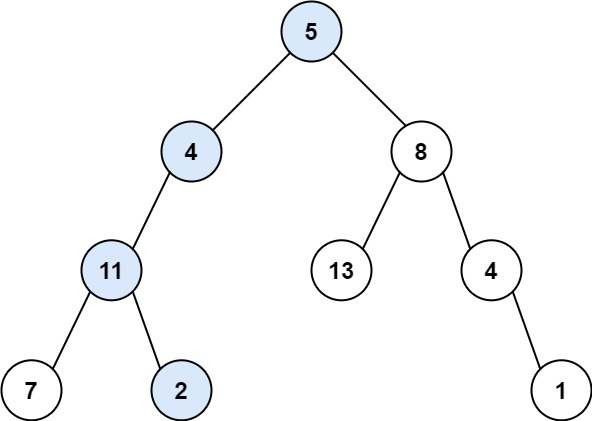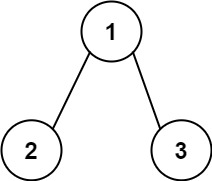# 112.path-sum

## Statement

• Difficulty: Easy
• Tag: `树` `深度优先搜索` `广度优先搜索` `二叉树```````输入：root = [5,4,8,11,null,13,4,7,2,null,null,null,1], targetSum = 22

````````````输入：root = [1,2,3], targetSum = 5

(1 –> 2): 和为 3
(1 –> 3): 和为 4

``````输入：root = [], targetSum = 0

``````

• 树中节点的数目在范围 `[0, 5000]`
• `-1000 <= Node.val <= 1000`
• `-1000 <= targetSum <= 1000`

• Difficulty: Easy
• Tag: `Tree` `Depth-First Search` `Breadth-First Search` `Binary Tree`

Given the `root` of a binary tree and an integer `targetSum`, return `true` if the tree has a root-to-leaf path such that adding up all the values along the path equals `targetSum`.

A leaf is a node with no children.

Example 1:``````Input: root = [5,4,8,11,null,13,4,7,2,null,null,null,1], targetSum = 22
Output: true
Explanation: The root-to-leaf path with the target sum is shown.
``````

Example 2:``````Input: root = [1,2,3], targetSum = 5
Output: false
Explanation: There two root-to-leaf paths in the tree:
(1 –> 2): The sum is 3.
(1 –> 3): The sum is 4.
There is no root-to-leaf path with sum = 5.
``````

Example 3:

``````Input: root = [], targetSum = 0
Output: false
Explanation: Since the tree is empty, there are no root-to-leaf paths.
``````

Constraints:

• The number of nodes in the tree is in the range `[0, 5000]`.
• `-1000 <= Node.val <= 1000`
• `-1000 <= targetSum <= 1000`

## Solution

``````# Definition for a binary tree node.
# class TreeNode:
#     def __init__(self, val=0, left=None, right=None):
#         self.val = val
#         self.left = left
#         self.right = right
from typing import Optional

class Solution:
def __init__(self):
self.res = False

def dfs(self, rt: TreeNode, sum: int) -> None:
if not rt:
return

sum += rt.val

if not rt.left and not rt.right:
if sum == self.target:
self.res = True
return

self.dfs(rt.left, sum)
self.dfs(rt.right, sum)

def hasPathSum(self, root: Optional[TreeNode], targetSum: int) -> bool:
self.target = targetSum
self.dfs(root, 0)
return self.res
``````